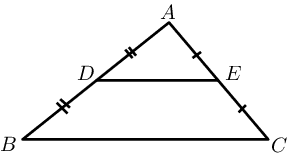Home Practice
For learners and parents For teachers and schools
Textbooks
Full catalogue
Pricing SupportLog in

We think you are located in United States. Is this correct?

# Chapter 8: Euclidean geometry

## 8.1 Revision (EMBJ6)

### Parallelogram (EMBJ7)

• Discuss terminology.
• Converses are not examinable.
• Good practice for answering questions:
• make a neat and accurate drawing
• state triangle/figure being considered
• give statement and appropriate reason
• give a conclusion

A parallelogram is a quadrilateral with both pairs of opposite sides parallel.

Summary of the properties of a parallelogram:

• Both pairs of opposite sides are parallel.

• Both pairs of opposite sides are equal in length.

• Both pairs of opposite angles are equal.

• Both diagonals bisect each other.

temp text

### The mid-point theorem (EMBJ8)

The line joining the mid-points of two sides of a triangle is parallel to the third side and equal to half the length of the third side.Given: $$AD = DB$$ and $$AE = EC$$, we can conclude that $$DE \parallel BC$$ and $$DE = \frac{1}{2}BC$$.

temp text